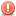CodeGuru Home VC++ / MFC / C++ .NET / C# Visual Basic VB Forums Developer.com

# Thread: Given an array that contains numbers from 1 to 1+n

1.Junior MemberJoin Date
Dec 2015
Posts
3

##Given an array that contains numbers from 1 to 1+n

Hello I have to do a task, but not sure how to proceed please could someone help me I would be really thankful so the thing I have to do is the following:

You are given an array A of size n that contains numbers in the range
1 to n + 1. We know that each number occurs at most once in A. Because there are only n
numbers in A out of n + 1 possible numbers, exactly one of the numbers is missing.

(a) Give a worst-case O(n) time algorithm that determines the missing number. Also prove
the running time of your algorithm.

(b) If the array A was sorted, we could find the missing number in O(log n) time instead.
Give an algorithm that finds the missing number in a sorted array in O(log n) time and
also prove its running time.Reply With Quote

2.Elite Member Power PosterJoin Date
Aug 2000
Location
West Virginia
Posts
7,717

## Re: Given an array that contains numbers from 1 to 1+n

I assume that this is homework, so I'll just give you some hints:

For (a) : use the fact that : 1 + 2 + 3 + ... + (n+1) = (n+1)*(n+2)/2

For (b) : do a binary search. Compare the array value at the current "mid" index
with the index itself.Reply With Quote

3.Junior MemberJoin Date
Dec 2015
Posts
3

## Re: Given an array that contains numbers from 1 to 1+nOriginally Posted by Philip NicolettiI assume that this is homework, so I'll just give you some hints:

For (a) : use the fact that : 1 + 2 + 3 + ... + (n+1) = (n+1)*(n+2)/2

For (b) : do a binary search. Compare the array value at the current "mid" index
with the index itself.
Thank you for the replay that hhelped me alot I think I have now and idea how to do the (b), but still can't figure out the first one. I am still new to algorithms and have some omissions in my knowledges.Reply With Quote

4.Elite Member Power PosterJoin Date
Aug 2000
Location
West Virginia
Posts
7,717

## Re: Given an array that contains numbers from 1 to 1+n

Say N = 10 .. The array will have the numbers 1 thru 11 (with one missing)

1 + 2 + 3 + ... + 11 = 11(12)/2 = 66

Sum the elements in your array. It will be less that 66, since one number is missing.Reply With Quote

5.Junior MemberJoin Date
Dec 2015
Posts
3

## Re: Given an array that contains numbers from 1 to 1+n

Thank you very much bro you helped me alot thumbs upReply With Quote

####Posting Permissions

• You may not post new threads
• You may not post replies
• You may not post attachments
• You may not edit your posts
•